# CBSE Class 12 Physics Chapter 9 Revision Notes

Chapter 9: Ray Optics and Optical Instruments Revision Notes

• Geometrical Optics or Ray Optics: Here light is viewed as a ray that travels in a straight line in this optics. It states that there is an image for each and every object.
• Reflection: The phenomenon of changing the path of light without changing the medium is known as reflection.
• Reflection of light occurs when light returns to the same medium from which it came after striking a surface.

## Laws of Reflection

The following are two reflection laws:

• The incidence angle I is equal to the reflection angle r, i.e. ∠I = ∠r.
• At the point of incidence, the incident ray, reflected ray, and normal to the reflecting surface are all in the same plane.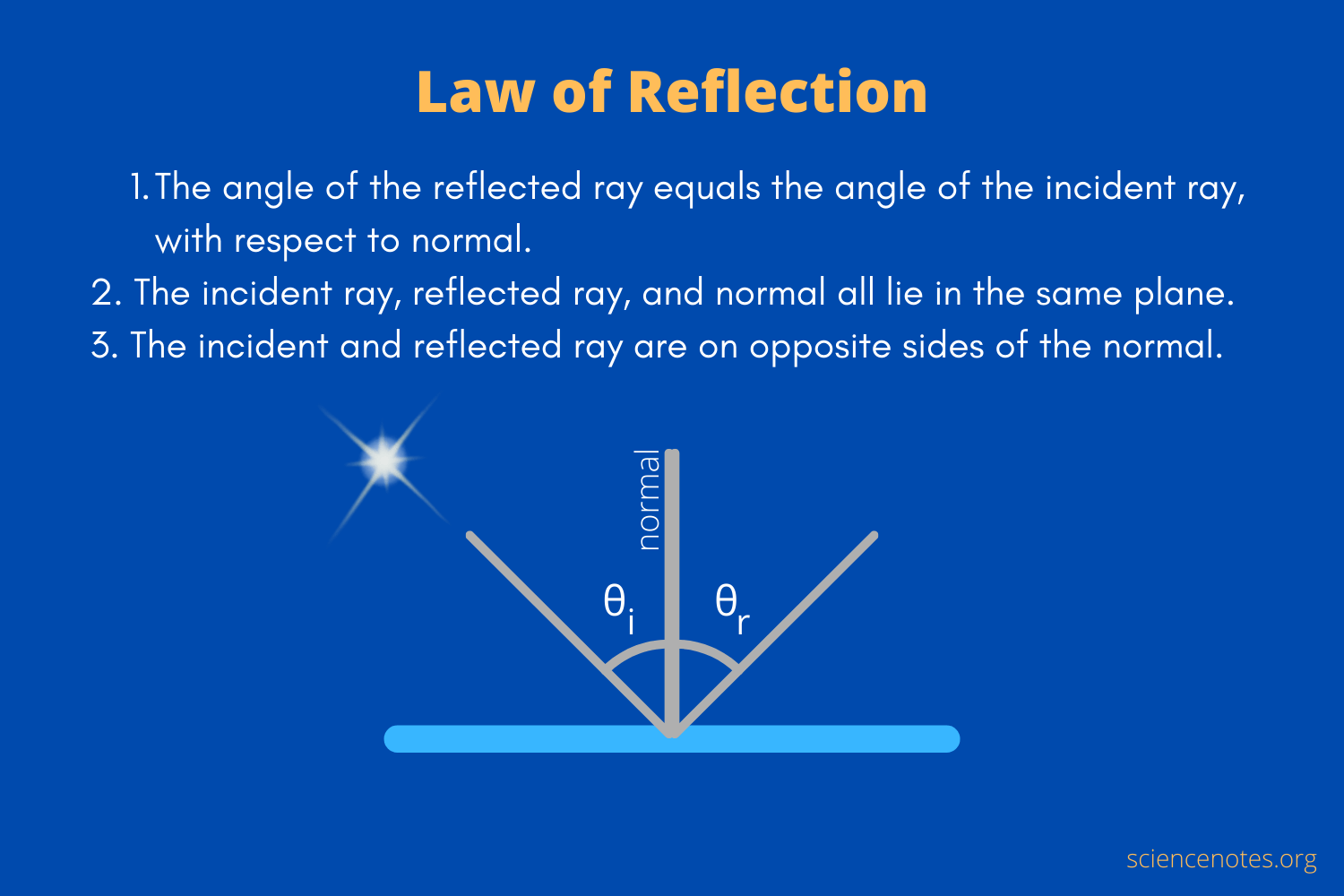Source

• Total number of images formed by two plane mirrors inclined at an angle θ with each other is given by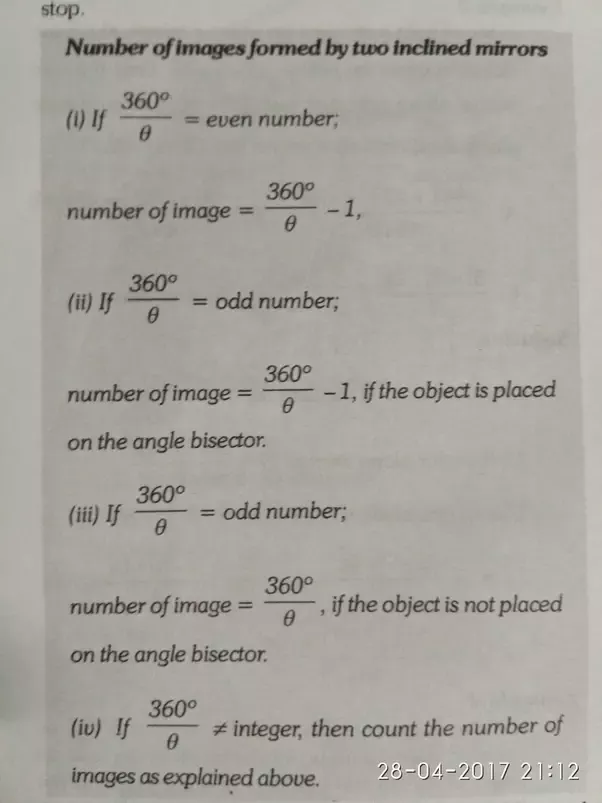Source

• A hollow sphere contains the reflecting surface of a spherical mirror. There are two types of spherical mirrors; concave spherical mirrors and convex spherical mirrors.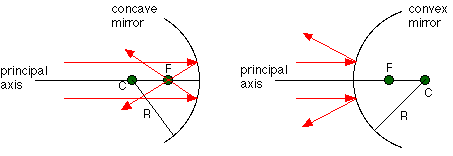Source

## Sign Conventions

• All measurements must be taken from the mirror’s pole.

• All measurements taken in the direction of the incident ray will be positive, while those taken in the opposite direction will be negative.

• All distances above the principal axis are measured as positive, and all distances below the principal axis are measured as negative.

• For a real object, u is negative, whereas for a real image, v is negative and for a virtual image, it is positive.

• Mirror Formula: is a relationship between the mirror’s focal length and the distances between objects and their images.

• Magnification in a straight line: The linear magnification produced by a spherical mirror is defined as the ratio of the size of the image formed by the spherical mirror I to the size of the object O.

• Magnification (m): It has a negative value for the real image and a positive value for the virtual image.

## Refraction

• Refraction is the phenomenon of light changing its path as it travels from one medium to another.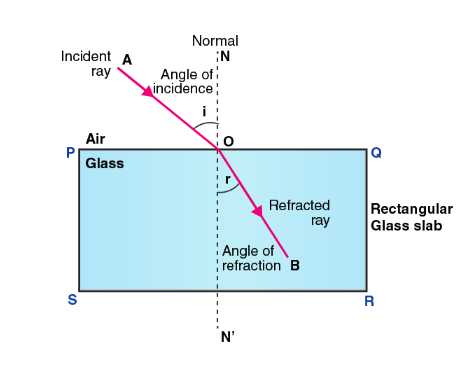Source

### Refraction Laws

The following are two refraction laws:

(i) At the point of incidence, the incident ray, refracted ray, and normal to the refracting surface are all in the same plane.

(ii) For the two given media, the ratio of the sine of the angle of incidence to the sine of the angle of refraction is constant. The relative refractive index, denoted by the letter n, is a constant.

When the first medium is air, n = sin i/sin r (Snell’s law), where n is the refractive index of the second medium.

• When a ray of light traveling from a denser medium to a rarer medium strikes the interface of the two media at an angle greater than the critical angle for the two media, the ray is totally reflected back to the denser medium. Total Internal Reflection is the name for this phenomenon.

• It only happens when the angle of incidence in the denser medium is greater (not equal) to the critical angle, i > ic.

• According to the principle of reversibility of light, when the final path of a ray of light after any number of reflections and refractions is reversed, the ray retraces its entire path.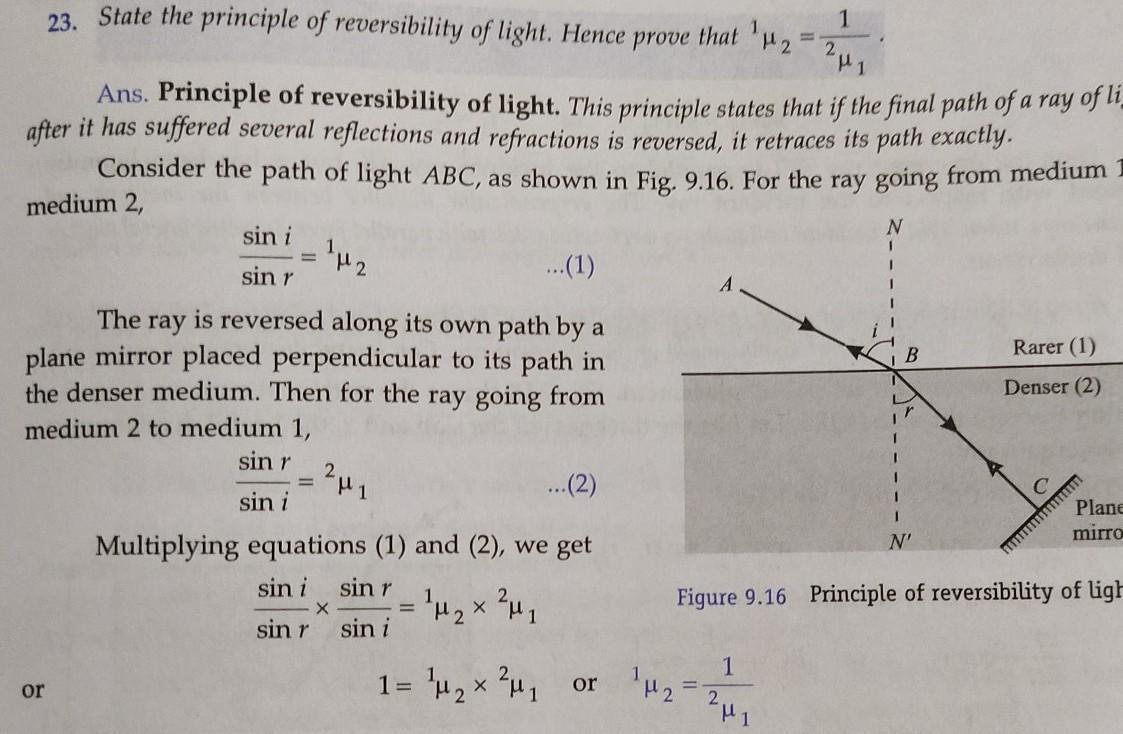Source

• Total internal reflection is used in optical fiber, mirage, diamond gleaming, and totally reflecting prisms, among other things.

## Lenses

• A lens is a transparent medium that is bounded by two spherical surfaces, one or both of which are spherical.
• Convex or converging lenses: A convex lens is one that is thicker in the center and thinner at the edges.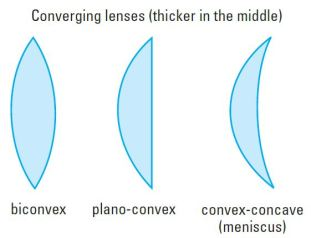Source

• Concave or diverging lenses: A concave lens is one that is thinner in the middle and thicker at the ends.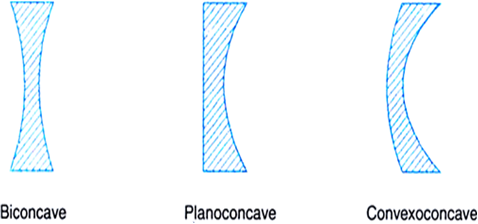Source

• The power of a lens refers to its ability to converge or diverge the rays of light that strike it.

• Magnification by lens combination m = m1 m2 m3………..

## Prisms

• Prisms have the ability to bend incident light towards their base.
• A prism is a portion of a transparent medium that is bounded by two plane faces that are inclined at a suitable angle to each other.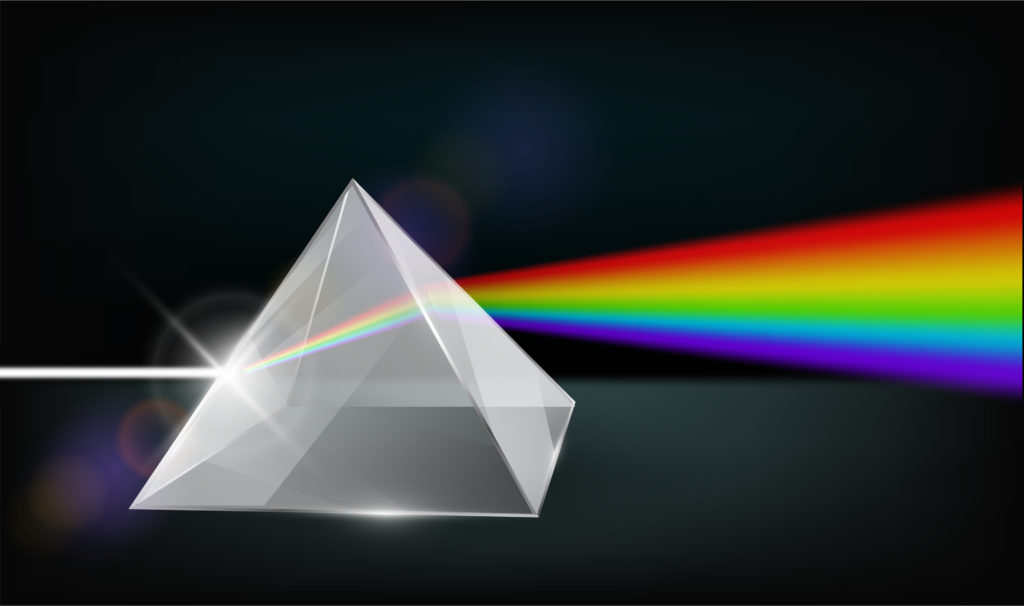Source

• If the prism is adjusted to the minimum deviation angle, the angle of incidence equals the angle of emergence.
• Prism-based dispersion: Dispersion is the phenomenon of light splitting into its component colors.
• Angular Dispersion is a term that refers to the dispersion of The difference in the angles of deviation for two extreme colors, violet and red, produced by a prism for white light is known as angular dispersion.
• Power of Dispersion: The ratio of angular dispersion to the mean deviation produced by a prism is known as its dispersive power.
]]>